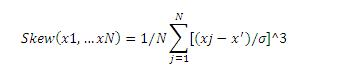# Skewness

Posted in Statistics, Total Reads: 2367

## Definition: Skewness

Skewness represents the degree of asymmetry of a distribution of values around its mean value and it is non-dimensional unlike mean or standard deviation. It characterizes the shape of the distribution and is denoted as:Where σ=σ ( is the standard deviation of the distribution. It describes which side of the distribution has a longer tail. Positive values of skewness denotes that the distribution has an asymmetric tail extending out more towards the positive , while negative values of skewness denotes that the distribution has an asymmetric tail extending more towards the negative  If the skewness value is zero, then it denotes that the distribution is symmetrical.

Steps for calculating the skewness are:

1. The mean and the standard deviation are calculated first
2. The mean is then subtracted from each value and the result is raised to the power of 3
3. The cubed deviations are then added
4. The value of (number of scores-1) is then multiplied with the cubed standard deviation
5. Finally the skewness is calculated by dividing step3 by step4.

Right skewness is obtained when the variable is left bounded like durations (response time etc.) or financial variables (income, prices etc.). Left skewness is obtained when the variable is right bounded i.e., when the variable has a specified maximum value like (scores in an exam, number of wickets in a cricket match etc.).

Hence, this concludes the definition of Skewness along with its overview.

Browse the definition and meaning of more terms similar to Skewness. The Management Dictionary covers over 7000 business concepts from 6 categories.

Search & Explore : Management Dictionary

Similar Definitions from same Category: